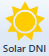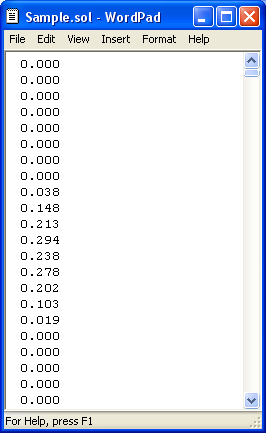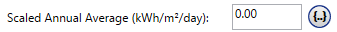# HOMER Pro 3.9

 Navigation: Design > Resources Tab Solar DNI ResourceThe Solar Direct Normal Irradiance (DNI) resource is used to calculate concentrating PV output. Concentrating PV uses optics to concentrate the solar radiation in a way that captures only the direct beam radiation (also called DNI) and not the diffuse or reflected components of the incident solar radiation.

Note: For a flat panel PV, enter a Solar GHI resource, not DNI. Flat panel PV captures direct, diffuse, and reflected radiation, and so uses the Solar GHI resource to calculate output.

The Solar DNI Resource page can be found under the Resources tab at the top of the page.

## Choose Locale

You can select a location on the map on the Home page by clicking the location on the map.

## Importing Solar Data

The Solar DNI resource can only by specified by importing a time-series data file. See the Finding data to run HOMER section of the help for a list of sources for solar data.

You can prepare your own text file containing solar radiation data in each time step for a complete year.

Tip: You can import data with any time step down to one minute. HOMER detects the time step when you import the data file. For example, if the data file contains 8760 lines, HOMER assumes it contains hourly data. If the data file contains 52,560 lines, HOMER assumes it contains 10-minute data.

The data file must contain a single value on each line, where each line corresponds to one time step. Each value in the file represents the average solar radiation (in kW/m2) for that time step. The first time step starts at midnight on January 1st. The following is a sample input file.Clickto open the text file. You can import a text file with any extension.

When you import data from a text file, HOMER makes a copy of the data set and integrates it with the HOMER (.homer) file. After the data is part of the HOMER file, HOMER no longer refers to the original text file. If you modify data in the original file, you must import the modified file for the modification to be included in the HOMER file. After you import a data file, HOMER calculates monthly average radiation and clearness index values and displays them in the table and graph. HOMER also displays the name of the imported data file in the title of the graph.

If you enter new monthly solar radiation values after importing data from a file, HOMER discards the data from the imported file and synthesizes new data based on the twelve new monthly averages. You can edit synthesized data by changing values in the solar resource table. To edit values from an imported file, you must edit the file directly and then import the modified file, as described above.

## Scaled Data for Simulation

HOMER uses scaled data for calculations. To create scaled data, HOMER multiplies each of the values in the baseline data by a common factor that results in an annual average value equal to the value that you specify in Scaled Annual Average.To determine the value of this factor, HOMER divides the scaled annual average by the baseline annual average solar radiation. The scaled data retains the shape and statistical characteristics of the baseline data, but may differ in magnitude. The default value for the scaled annual average is the baseline annual average solar radiation. When the two values are equal, the scaled data and baseline are identical. HOMER interprets a scaled annual average of zero to mean that there is no solar radiation.

Two reasons to use a scaled annual average that is different from the baseline annual average are for unit conversion and sensitivity analysis.

An example of using the scaled annual average for unit conversion is to convert data from an imported file that contains solar radiation data expressed in Wh/m2 rather than kWh/m2. If the baseline annual average is 4800 Wh/m2, you can enter 4.8 in Scaled Annual Average, so the scaled data is equivalent to the baseline data, but expressed in kWh/m2: 1 kWh/m2 = 1000 Wh/m2.

Note: To the right of the Scaled Annual Average input is a sensitivity button () that allows you to do a sensitivity analysis on that variable. For more information, see Why Would I Do a Sensitivity Analysis?